Next: Bibliography Up: Interconnect-aware Low Power High-level Previous: Acknowledgments

# Appendix

The fixed-taper buffer structure  is commonly used for output buffer design. It consists of a series of inverters, in which each inverter is larger than its upstream neighbor by the same factor. From , the dynamic power dissipation of the entire tapered buffer system is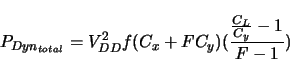where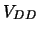is the supply voltage,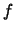the frequency,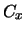the output diffusion capacitance of the first inverter,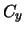the input gate capacitance of the first inverter,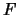the fixed taper factor, and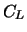the load capacitance.consists of two components,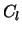, the downstream gate input capacitance which is the real load, and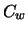, the capacitance of the wire connecting the driver and the load. Then the total capacitance is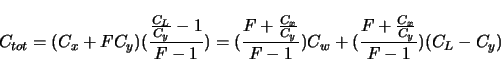The second term on the right is independent of the interconnect. It can be incorporated into the input capacitance of the corresponding downstream DPU(s). The power consumption due to it can be taken into consideration by the DPU's power model. Therefore, we only need to worry about the first term when computing interconnect-related power consumption.is equal to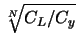, where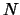is the number of inverters in the buffer, or, stages in the buffer. For power-delay product minimum buffers,ranges from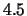to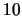.is usually smaller than. Thus, we can estimate the interconnect-related equivalent capacitance of the buffer,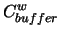, as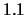to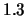times the wire capacitance. In our estimation, we assume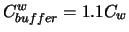. Hence, the interconnect-related dynamic power dissipation of the buffer is abouttimes that of the metal wire.Next: Bibliography Up: Interconnect-aware Low Power High-level Previous: Acknowledgments
Lin Zhong 2003-10-11